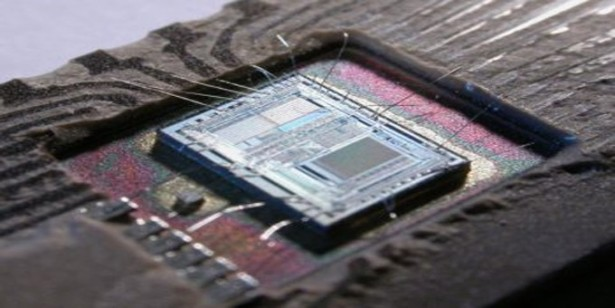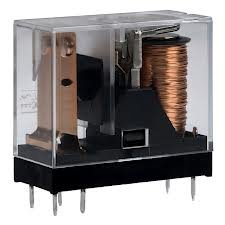# How-to use PWM to Generate Analog (or Analogue) Voltage in Digital Circuits

Many times, designers are faced with the need of generating analogue or analog voltage levels in purely digital circuits. Although the market provides today a very broad range of dedicated digital-to-analogue converters, putting such a device in the schematic has a negative impact on the overall cost of the system.

### 1. Introduction

In the first part of this article (Generating Analog Voltage with Digital Circuit (I) ), we have detailed what is known as the R2R method for obtaining an analogue voltage. The advantage of this method is that it is very much similar to use a real off-the-shelf DAC integrated circuit; the digital values you would need to generate with the code running on the microcontroller are the same in both cases.
The main drawbacks of this method, however, is the high number of pins required on the microcontroller (directly proportional to the resolution you need) and the relatively high number of external components needed (an additional pair of resistors for each additional bit of resolution).
In order to address these problems, another solution is available: the PWM solution. This allows us to obtain analogue voltage using just one pin of the microcontroller, and as little as two external components (figure 1).The theory behind the solution is that if you have low pass filter good enough and a pulse train signal, you will be able to obtain at the output of the filter the medium value of this particular signal; the medium value of a pulse train signal is always proportional to the duty cycle of the pulse train. If your duty cycle is 0%, then your PWM signal is actually always 0, and the signal at the output of the filter will obviously be also 0. If the duty cycle is 100%, then your PWM signal is actually a continuous voltage signal, equal to VCC, which even filtered, will yield the same continuous value: VCC.
In case the duty cycle is varied between these two limits (0% and 100%) the low-pass filter (provided it is correctly dimensioned) will basically cut the variations in the signal, leaving only the continuous component of the signal (its medium value) pass through. The diagrams in the figure 2 and 3 illustrate the case in which the duty cycle is 50% and 10% respectively. The green represents the PWM signal, while the red represents the continuous (analogue) signal obtained at the output of the filter.

READ  PIC18 Pulse Width Modulation (PWM) DC Motor Speed Controller with the RPM Counter Project